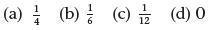# Chapter 8 – Statistics

Consider the following statements and click to reveal the answer.

1. Decide whether these groups of data are discrete or continuous:

(a) The population of a city.

(b) A child’s height.

(c) The speed of a car travelling along the motorway.

(d) The frequency of the word ‘broomstick’ in a Harry Potter book.

(a) discrete (b) continuous (c) continuous (d) discrete

2. A scattergraph showed a good positive correlation between the number of storks seen nesting in a town and the number of babies born. What does this tell you about the possibility of ‘storks delivering babies’?

Nothing. Have you heard the saying, ‘Lies, damned lies and statistics’? This is a good example of how data may have shown a correlation but in fact does not prove that babies are brought by storks! This is pure coincidence!

3. The following are two sets of marks from the same group of children. The first set is from a mathematics test and the second from an English test. Both tests were marked out of 20. The marks have been put in order for you.

Maths: 5, 7, 8, 8, 9,10, 10, 11, 12, 12, 12, 12, 13, 13, 14, 15, 15, 15, 16, 16, 17, 17, 17, 18, 18, 18, 18, 19, 19, 20
English: 3, 5, 5, 7, 8, 8, 9, 9, 10, 10, 10, 10, 11, 12, 12, 13, 13, 14, 14, 14, 14, 14, 15, 15, 16, 17, 17, 17, 18, 19

(a) Which test did the children tend to score better in? How do you know?
(b) Calculate the mean, median and mode for each set of data if you have not already done so.
(c) Work out the range and interquartile range for both sets of data.
(d) What reasons might you think of to explain the difference in test scores?

(a) Maths. Reasons for choice will vary, but may be based on answers (b) and (c).

(b) Maths: mean = 13.8 median = 14.5 mode = 12,18

English: mean = 12 median = 12.5 mode = 14

(c) Maths: range is between 5 and 20

interquartile range is between 10.5 and 17.5

English: range is between 3 and 19

interquartile range is between 9 and 15

(d) The only valid statement we can make is that the maths scores were higher than the English scores. It is impossible to tell from the data pre­sented why this might be, but some possible reasons could be:

• the English test was harder than the maths test;
• the pupils are better at maths than they are at English;
• the English test was taken in poor conditions;
• some of the pupils cheated in the maths test!

4. Consider the throwing of a regular six-sided die and the tossing of a coin. What is the probability that:

(a) an even number and a head are shown?
(b) a multiple of 3 and a tail are shown?
(c) a 1 and a head are shown?
(d) a multiple of 7 and a head are shown?5. List four ways of displaying discrete data.

Four ways of displaying discrete data are (any four of) table, block graph, bar graph, bar-line graph, pie chart, scattergraph.

6. List four ways of displaying continuous data.

Four ways of displaying continuous data are scattergraph, line graph, fre­quency diagram, frequency histogram (possibly with a cumulative frequency diagram/curve).

7. Define the following terms:

(a) Mean
(b) Median
(c) Mode
(d) Range
(e) Quartiles

(a) Mean – the most common type of average; found by adding up all of the components in a set of data and dividing by the number of components in the set.
(b) Median – the middle value in a set of data.
(c) Mode – the value occurring most commonly in a set of data.
(d) Range – a measure of the spread of a set of data: the interval between the least/greatest values.
(e) Quartiles – another way of measuring a set of data: the lower quartile is the value one quarter of the way along a set of ordered data; the upper quartile is the value three quarters of the way along that set of ordered data.

8. What are the three possible ways of identifying the probability of an event?